\$B:#>,\$N0lGU(B

 1(I=L_X]8^%?]8^(B 2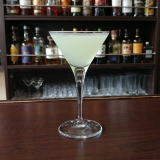(I=L_X]8^%L(0X]8^(B 3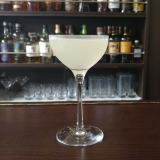(IWJ^0=^%9^0Q(B 4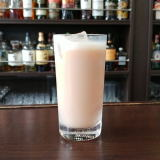(IJ^@LW2(B 5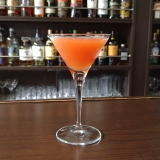(IRX0%<^*0](B 6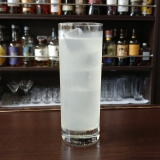(I41R0Y(B 7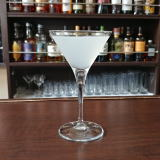(IW2W(B 8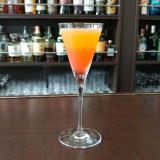(I:^0YC^]%D^0](B 9(IWQ%:08(B 10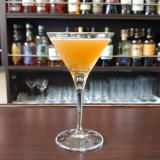(IS]C6Y[(B 11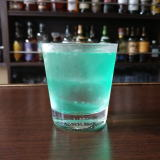(I1L@0%P/D^E2D(B 12(IF-0%\0YD^(B 13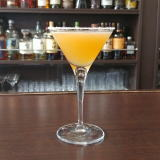(I8W 14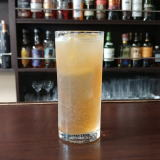(IWQ%J^/8(B 15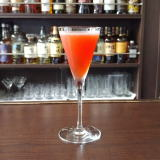(IZ/D^%6DZ1(B 16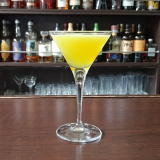(IWL^%=D0X0(B 17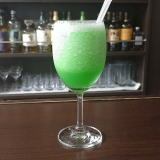(IJ\21]%@^]=(B 18(IJ^/D%J^2D(B 19(IL^W/8%J_/<.](B 20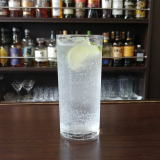(I35/6%DF/8(B 21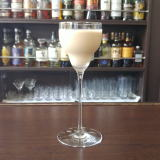(I506^=^Q(B 22 \$B5Y\$_(B 23(I34C^(]8^%M^Y(B 24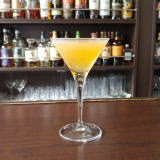(I?IW(B 25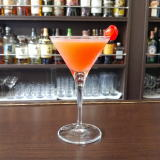(I6XK^1]%60FJ^Y(B 26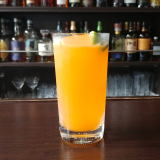(IO]N^(B5 27(IPX5]@^W0(B 28(I;]>/D%D^W2J^0(B 29(IC^-N^H(B 30(IJ/K_0%4S0<.](B